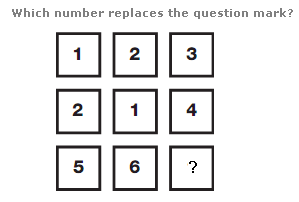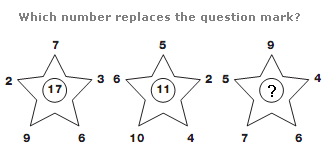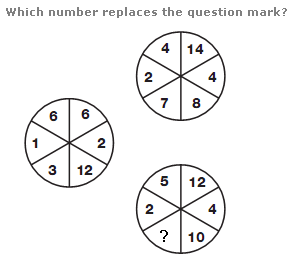# Puzzles - Number puzzles

### Exercise :: Number puzzlesAnswer : 3 Explanation : Reading each column as a 3 digit number, moving from left to right, the columns follow the sequence of cube numbers of 5, 6 and 7.Answer : 13 Explanation : In each star, the central value equals the sum of the upper and lower digits on each star, subtracting the sum of the left and right hand digits.Answer : 6 Explanation : In each circle, double the numbers on the left hand side and put the result in the opposite segment on the right hand side.